﻿
Reach Us+44-7482-875032
Application of Weighted Essentially Non-Oscillatory Scheme to Solve the Dusty-Gas Flow Model

# Journal of Applied & Computational MathematicsOpen Access

All submissions of the EM system will be redirected to Online Manuscript Submission System. Authors are requested to submit articles directly to Online Manuscript Submission System of respective journal.

# Application of Weighted Essentially Non-Oscillatory Scheme to Solve the Dusty-Gas Flow Model

Aslam RD1, Ali A1*, Rehman A1 and Qamar S1,2
1Department of Mathematics, COMSATS University Islamabad, Park Road Chak Shahzad, Islamabad, Pakistan
2Max Planck Institute for Dynamics of Complex Technical Systems, Sandtorstrasse, Magdeburg, Germany
*Corresponding Author: Ali A, Department of Mathematics, COMSATS University Islamabad, Park Road Chak Shahzad, Islamabad, Pakistan, Tel: +923216583406, Email: [email protected]

Received Date: Sep 09, 2017 / Accepted Date: Sep 20, 2018 / Published Date: Sep 27, 2018

Keywords: Dusty-gas flow; WENO finite volume scheme; Central upwind scheme; Contact discontinuity; Rarefaction wave; Shock wave

#### Introduction

The discipline over the mixture of fluids and dust particles is of great interest due to its extensive applications in astrophysical, underground explosions, fluidized beds, explosive volcanic eruptions and electrostatic precipitation of dust and geophysical problems, etc. [1-6]. The basic physics of these flows is sophisticated and so, it's troublesome to create a general approach that consolidates every phase of the flow. Therefore, these flows are classified into several phases i.e., the fluidization stage, the porous medium phase, the slugging phase, the sedimentation stage and the flow of dusty-gas mixture, [7-10]. Here, our concern is the mixture of gas and dust particles which is one of the tremendous sort of two-phase flow, in which the dust particles occupy less than 5% of the entire volume. Therefore, when the velocity of gas is extremely high then these dust particles are treated as pseudo-fluid. So, the dusty-gas mixture is considered as a mixture of two fluids. In order to preserve an equilibrium flow condition, it is assumed that the velocities of gas, solid-particles and of the mixture are same in the flow field. Furthermore, it is also assumed that the temperature of gas, solidparticles and of the mixture are the same. First, Engel and Steenbeck  give an introduction of the temperature as well as diameter of solid-particles for improving the basic equations of solid-gas mixture, by considering insignificantly small volume fraction of solid-particles in the mixture. Goldsworthy  then extract the basic equations for the mixture of solid-gas by using the theory of continuum and also, taken under consideration the volume fraction of the solid-particles. For more details on the basic equations for solids and gas mixture [13-16]. Subsequently, Pai  elaborates the basic equations of the mixture of solid and gas, both microscopically and macroscopically. Furthermore, he extracted these model equations using the Boltzmann's transfer equations of mixture to obtain a greater perception of the internal energy and the partial pressure. Lastly, Gupta et al.  conservatively extract the basic equations of the solids and gas mixture and used the direct approach to find the solution for these model equations [18-20]. Recently, these model equations are calculated with the upwind CE / SE scheme .

In this paper, we are going to utilize the WENO finite-volume scheme to compute the model of dusty-gas flow, which is a completely different numerical scheme from the upwind CE/SE scheme . The WENO finite volume scheme was introduced [23,24]. Afterwards, the authors introduced a general method to construct the WENO schemes of arbitrarily higher order accurate . These schemes also handle the multi-dimensional problems [26,27] more precisely than the low order accurate schemes. The main advantage of higher order WENO schemes is to hold the non-oscillatory property near the discontinuities and at the same time it is capable to carry out the arbitrary high order accuracy near the smooth solutions. The flow of dusty-gas is complicated compared to the perfect gas flow. The mixture obeys different properties such as, heat transfer, sound speed etc., in the comparison of perfect gas because of the appearance of small solid-particles in the gas. We considered the dust particles of small size in the mixture to obtain the average properties of the dust particles. Several test problems are assumed to prove that the suggested scheme is extremely robust, works very well even in low density flows and offers better resolutions of sharp discontinuity. Finally, we compare the numerical results generated by the suggested scheme with the results generated by exact Riemann solver and that of well-known central-upwind scheme to validate the proposed scheme.

The rest of article is formulated as, the model of dusty-gas flow is presented. A short summary of the exact Riemann solution for the proposed model is presented. The construction of WENO finite-volume scheme for the proposed model is presented. Certain test problems are considered to compare the numerical results obtain by WENO scheme with those of central upwind scheme [28-30] and the exact Riemann solver. At the end, it presents the conclusions of article.

#### The Model of Dusty-Gas Flow

The governing equations for 1-D, time dependent flow of dusty-gas mixture are given as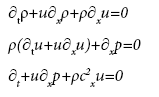(1)

where u represents the particle velocity along x-axis, the time is represented by t, the density is represented by ρ and the pressure is represented by p. The system in eqn. (1) agrees the Mie Gruneisen type equation of state which is expressed as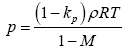(2)

Where the quantities M and kp represents the volume fraction and the mass fraction respectively, R represents the gas constant as well as T represents the temperature of mixture. The relation among the quantities M and kp is given as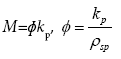(3)

Where ρsp represents the specific density of solid-particles. The internal energy/unit mass for the mixture is defined by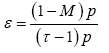(4)

Where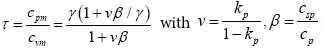and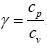Furthermore the quantities cvm and cpm represents the specific heats of the dusty-gas mixture at constant volume and constant pressure respectively. Also, the quantities cv and cp represents the specific heat of gas at constant volume and pressure respectively. The quantity csp denotes the speci c heat of solid-particles. The system of governing in eqn. (1) with eqn.(4) in conservative form are given as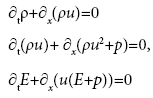(5)

Where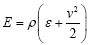and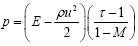. The system of eqns. (5) in compact form can be rewritten as

tw+∂xf(w)=0 (6)

With

w=(w1,w2,w3)T=(ρ,ρu,E)T (7)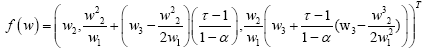(8)

Hence, all the primitive variables are obtained explicitly. The Jacobian matrix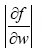has the following eigenvalues

λ1=u-c, λ2=u, λ3=u+c (9)

where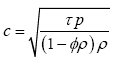is the equilibrium speed of sound.

#### Exact Solution for the Model of Dusty-Gas Flow

A short summary of exact Riemann solver for the model of dustygas flow is presented here. For further details about the exact Riemann solver [17-20,29,30] and references there in. The exact solution to the Riemann problem in eqns. (6,10)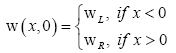(10)

has three waves associated with the eigenvalues in eqn. (9). These three waves divide the structure into four different constant states, and that are defined from left to right as: wL (known left data), wL∗(unknown left data), wR∗(unknown right data) and wR (known right data). The key step to solving the Riemann problem is finding the constant states wL∗ and wR∗ in the star region. In short, we need to evaluate the following physical quantities pL∗=pR∗=p,uL∗=uR∗=uL∗ and ρR∗. First we have to solve the non-linear algebraic equation for the pressure p to find all these quantities. The algebraic equation for p is given by

ϕ(p,wL,wR)=ϕL(p,wL)+ϕR(p,wR)+uR−uL=0. (11)

Here uL is the velocity on left state and uR is the velocity on right state, ϕL and ϕR are functions of connecting the left and right states respectively, to the unknown regions. The function ϕL is given byand the function ϕR is given by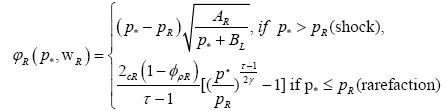The constant quantities AL, AR, BL and BR yields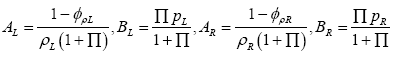(12)

Here,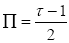Unlike the perfect gas dynamic, the solution within the rarefaction waves cannot be evaluated directly in case of dusty-gas flow. Therefore, we require an additional iteration procedure to evaluate the roots of nonlinear equation inside the rarefaction fan. This nonlinear equation with unknown p for the left rarefaction is given by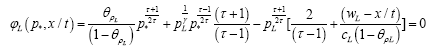(13)

and the nonlinear equation for right rarefaction is shown as(14)

The unknown value of pressure p in eqn. (11) or in eqn. (13) and (14) is obtained by Newton Raphson iterative procedure and the initial guess po to start a iterative procedure is obtained by using the arithmetic mean of the initial data for pressure, as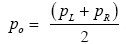. Once the value of pressure is known, then remaining unknown values are easily found by following the procedure as described for the ordinary gas dynamics .

#### Construction of WENO Finite-Volume Scheme for the Proposed Model

This section presents the construction of higher order WENO method finite-volume for the model of dusty-gas flow. The eqn. (6), we have

wt+f(w)x=0. (15)

The computational domain Ω is discretized with cells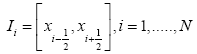the centre of the i-th cell is denoted by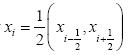and the size of i-th cell is denoted by Δxi. By integrating in eqn. (15) over the cell Ii, we obtained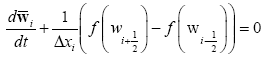where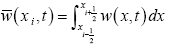. In order to obey upwinding for stability, we replace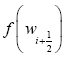by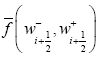and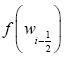by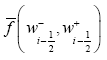we get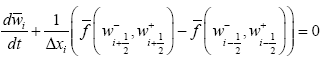(17)

In eqn. (17)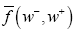represents the monotone numerical flux and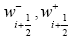are point wise approximation to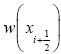. In this article, we utilize the Lax-Friedriches flux as a monotone numerical flux which is defined below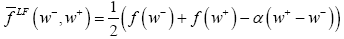Where α=maxw|f ’(w)|

Where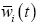are computational variables, which approximate the cell averages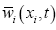. The point wise approximations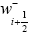and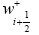are evaluated through the nearby cell average values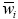by (2m-1)th order WENO reconstruction procedure as following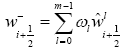(18)

The reconstruction to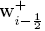is mirror symmetric of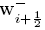. In this article we are considering fifth-order WENO finite –volume scheme ,so by setting m=3 in eqn. (18) gives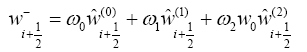(19)

where w0, w1 and w2 are non-linear weights. In eqn. (18),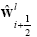are m reconstructed values of mth order accuracy and defined as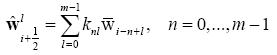(20)

corresponding to m different candidate stencils

Sn(i)={xi-n,…,xi-n + m-1}, n=0,…, m-1 (21)

The coeffcient knl gives guarantee that each m reconstructed values is mth order accurate, for detail . For m=3 in eqn. (20) becomes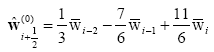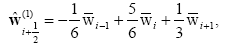(22)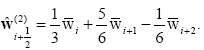The non linear weights w0, w1 and w2 in eqn. (19) are defined as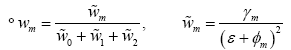(23)

where γm represents the linear weights, ε=10−6 and the smooth indicators ɸm for the 5th-order

WENO finite-volume scheme are defined as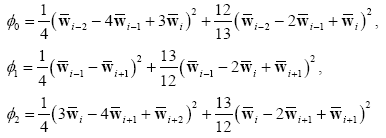(24)

The linear weights used in eqn. (23) are given as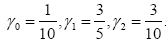(25)

The semi-discrete scheme in eqn. (17) is re-written as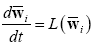(26)

In order to carry out third-order accuracy in temporal discretization, the 3rd-order TVD Runge Kutta time discretization scheme , for more details [32-34], is applied to solve in eqn. (26) as follows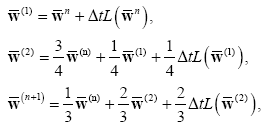(27)

where L(w) is the spatial operator and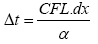with α=max(w|λ(w)|).

#### Numerical Test Problems

In this section, five 1-D numerical test problems are presented. The comparison of results obtained by WENO finite-volume scheme, central upwind schemes and the exact Riemann solver is given. As mentioned before, the solid-particles occupies only less than 5% of the total volume of mixture. Therefore, we need to choose the befitting value of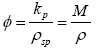(where ρ=max(ρLR)) so that the volume fraction M remains less than 5% on both sides of initial discontinuity. All the numerical test problems admit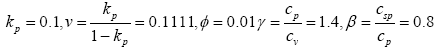and the collision of two shocks problem having ɸ=0.001667. In all test problems the computational domain for both numerical WENO finitevolume and central upwind schemes is divided into 200 mesh cells.

Problem 1: The shock tube problem , with the following initial data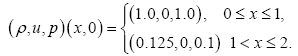The purpose of this problem is to access the entropy satisfaction property of the suggested numerical scheme. The computational domain is used to be [0,2] at t=0.12 as final time and the numerical results are exposed in Figure 1. The comparison shows that both WENO scheme and central-upwind scheme give correct positions of discontinuities and an oscillation free pressure solution can be observed. However, it can be seen that WENO scheme resolves sharp discontinuities better than central-upwind scheme. Also the results computed by the WENO scheme are very close to the results of exact Riemann solver.

Problem 2: A 123 test problem , that is also known as internal energy and low density problem. The problem having the following initial states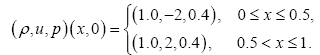This numerical test problem is used to analyze the ability of suggested numerical scheme for resolving contact discontinuity at low density. The solution profiles in computational domain [0,1] at t=0.15 are exposed in Figure 2 show that the suggested numerical scheme is more accurate than that of central-upwind scheme in term of resolving the contact discontinuity. The solution consist of two strong rarefaction wave thats why this problem is also known as strong rarefaction problem.

Problem 3: The left half of the blast problem, with the following initial data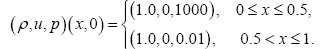This test problem was introduced by the Woodward and Colella . The pressure of the mixture is larger on the left data state than those of right data state. The main objective of the problem is to analyze the accuracy and robustness of the suggested numerical scheme. The computational domain used [0,1] is discretized into 200 mesh cells. The numerical results at t=0.012 displayed in Figure 3 show that there is a good agreement between the results generated by the WENO scheme and the exact Riemann solve. But we observe that central upwind scheme does not resolve contact discontinuity sharply in density profile as compare to WENO scheme.

Problem 4: The collision of two shocks problem. The initial state is given below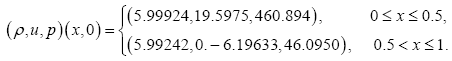In this test problem we set ɸ=0.001667, the computational domain used [0,1] is discretized into 200 mesh cells. The numerical results at t=0.035 displayed in Figure 4 show that the suggested scheme resolve the contact discontinuity superior than those of central upwind scheme. Also the results evaluated by the suggested scheme are very close to the results of exact Riemann solver. The solution incorporates the two strong shocks and a contact discontinuity.

Problem 5: The Stagnant contact discontinuity problem. The initial state is given below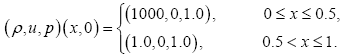The computational domain used [0,1] is divided into 200 mesh point at final time t=10. In this test problem density on left data state is larger than those on right data state while pressure and velocity is same on both states causing a uniform constant state and there is no oscillation. In density profile, we observe that the stagnant contact discontinuity is sharply captured by the WENO scheme than those of central-upwind scheme displayed in Figure 5.

#### Conclusions

In this paper, the WENO finite-volume scheme was utilized for model of dusty-gas flow to get the numerical results. The fifth-order WENO scheme was used for the spatial reconstruction and for the temporal discretization. The 3rd-order TVD Runge-Kutta algorithm was employed. Different test problems were considered to analyze the performance of proposed numerical scheme. The results obtained by suggested numerical scheme were compared with those of exact solutions and the central upwind scheme. The proposed numerical scheme preserved the non-oscillatory property near strong discontinuities. We observed that the WENO scheme efficiently resolved the contact discontinuities and most importantly it preserved the positivity of flow variables. Comparatively, the proposed numerical scheme produced better results than the central upwind scheme. An amazing agreement was noted among the solutions of suggested numerical scheme and exact Riemann solver.

#### References

Citation: Aslam RD, Ali A, Rehman A, Qamar S (2018) Application of Weighted Essentially Non-Oscillatory Scheme to Solve the Dusty-Gas Flow Model. J Appl Computat Math 7: 412. DOI: 10.4172/2168-9679.1000412

Copyright: © 2018 Aslam RD, et al. This is an open-access article distributed under the terms of the Creative Commons Attribution License, which permits unrestricted use, distribution, and reproduction in any medium, provided the original author and source are credited.

Select your language of interest to view the total content in your interested language

##### Recommended Journals
Viewmore
###### Article Usage
• Total views: 654
• [From(publication date): 0-0 - Aug 18, 2019]
• Breakdown by view type
• HTML page views: 606
• PDF downloads: 48

## Post your commentCan't read the image? click here to refresh
###### Peer Reviewed Journals

Make the best use of Scientific Research and information from our 700 + peer reviewed, Open Access Journals

International Conferences 2019-20

Meet Inspiring Speakers and Experts at our 3000+ Global Annual Meetings

Top